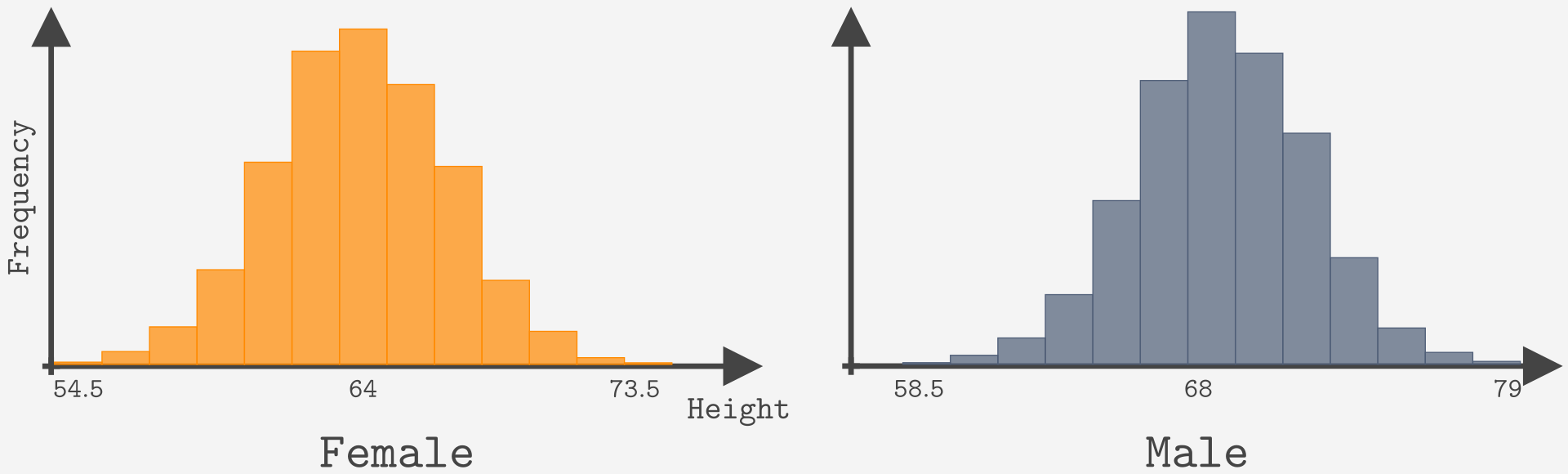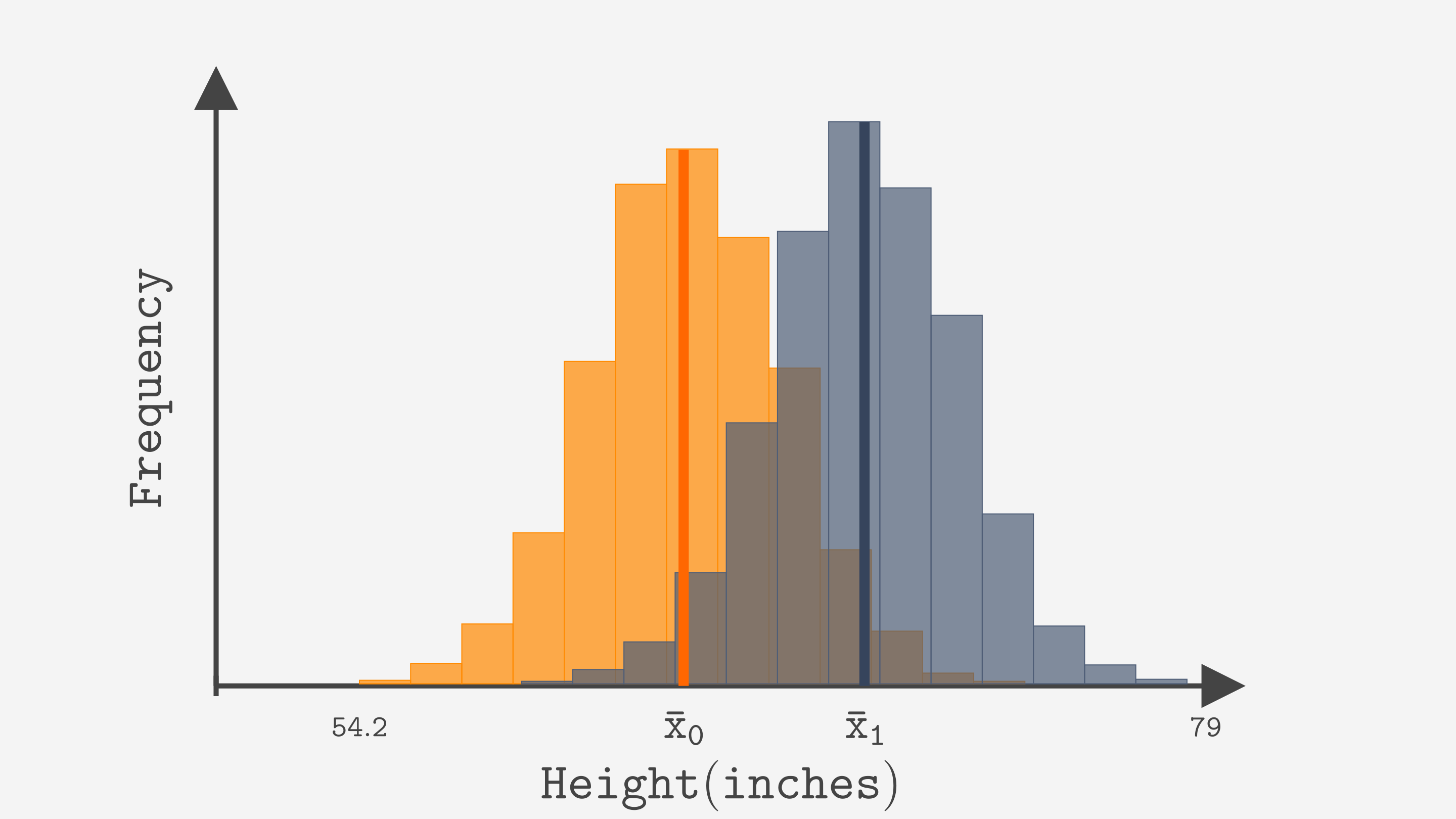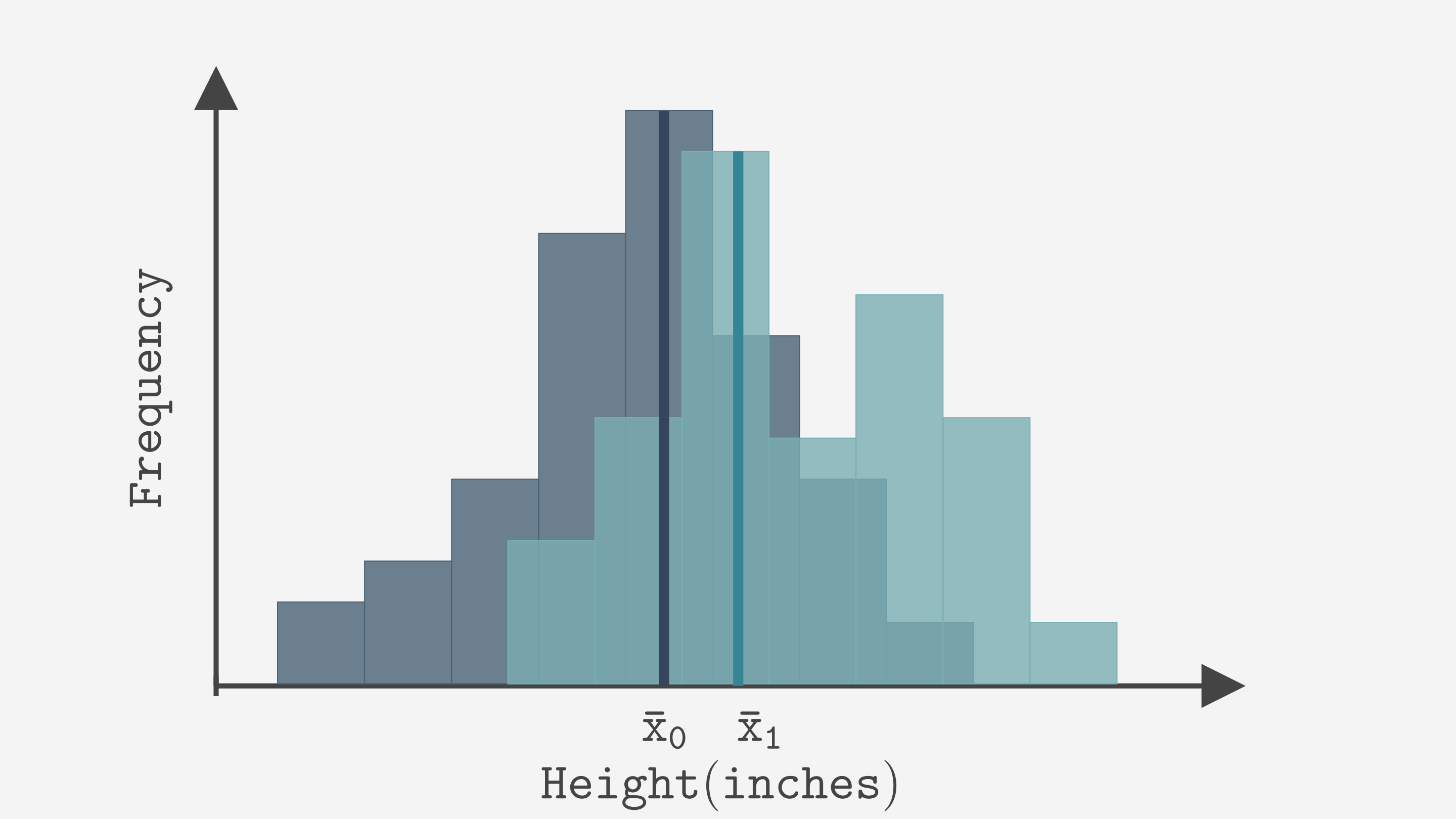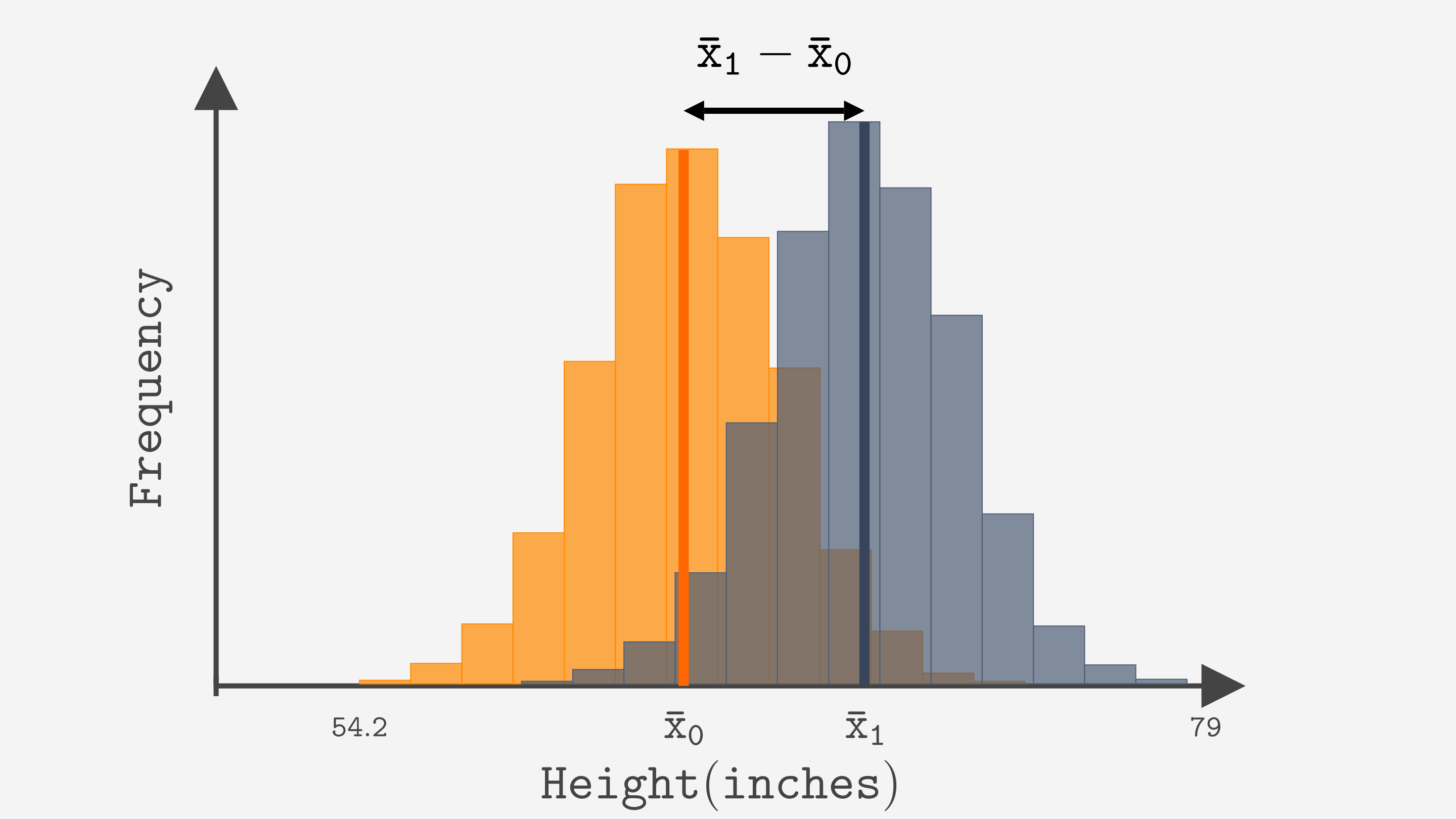Course Content

# Learning Statistics with Python

Learning Statistics with Python

##What is t-test

T-test is a statistical test used to determine whether there is a significant difference between the means of two samples.
To better understand the definition, let's look at the example.
Let's say we want to determine whether there is a significant difference between male and female height.

For that, we need two samples: male heights and female heights.Calculating the means and plotting the histograms together gives us this:Looking at that, we can state that males are taller on average. Mystery solved? Not quite. Let's look at one more example below.These are two samples of male heights. They are sampled from the same population and should be equal. But by chance, more tall men appeared in the second sample. We cannot state that men are taller than men. The difference between those two samples is purely the product of chance.
Coming back to our example male/female example.Is the mean difference here the product of chance too, or do samples indeed differ? That's precisely the question the t-test can answer.

Everything was clear?

Section 6. Chapter 1